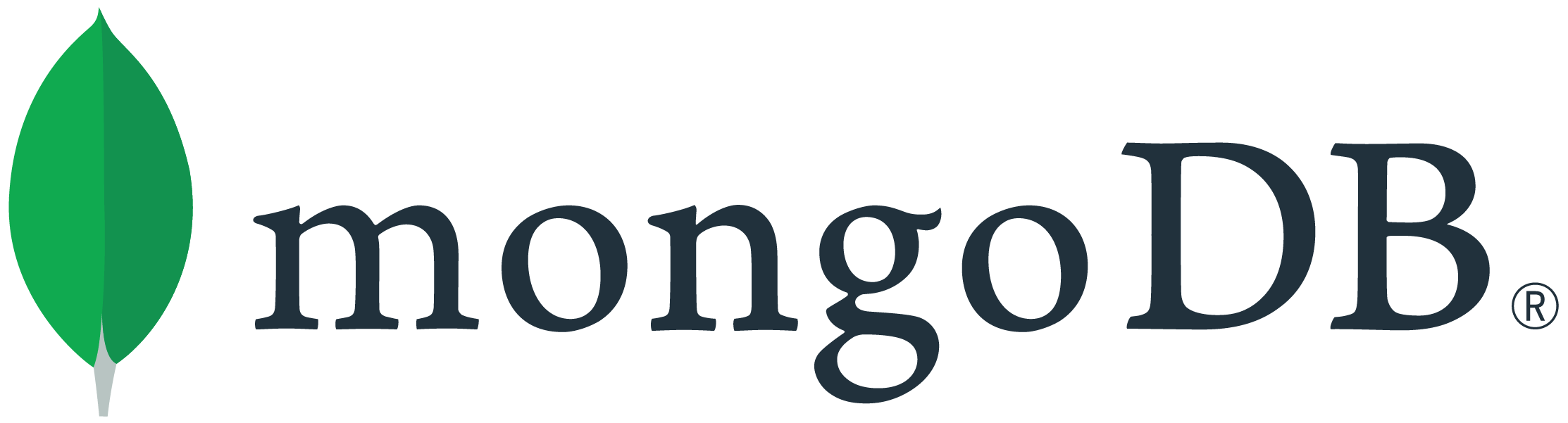# MongoDB C# driver deserialization introduce discrepancies

Hi folks,

I’m using c# 2.10.4 driver, and encountered data discrepancy using this code

``````    public void testDeserialization()
{
string jsonString = "{ a: 1, b: 0.5710711036366942 }";

BsonDocument doc = BsonDocumentSerializer.Instance.Deserialize(context);
Console.WriteLine(doc.ToString());
}
``````

This simple test code will output below, there is one more ‘2’ digit in the last!
{ “a” : 1, “b” : 0.57107110363669422 }

Seems this is related to double/decimal deserialization, any idea how to resolve this discrepancy?

Actually I’m using BsonDocument.ToJson() extension.

Console.WriteLine(doc.ToJson());

This will output the same results with discrepancy

Seems this is related to double/decimal deserialization,

I don’t think this is specifically related to the MongoDB C# driver, but instead due to the binary representation of double and float numbers in C#. To elaborate, C# represents double and float numbers in binary, and unable to represent many decimal numbers accurately. Depending on the requested precision of the `ToString()` conversion, the binary value would be to the closest binary equivalent.

To illustrate, see this simple code:

``````    var x = 0.5710711036366942D;
Console.WriteLine(x.ToString("G17"));
// outputs
// 0.57107110363669422
``````

any idea how to resolve this discrepancy?

If you have control over the input string to be parsed, you could explicitly express the decimal format with NumberDecimal(). For example, you could use

``````    string jsonString = "{ a: 1, b: NumberDecimal('0.5710711036366942') }";
...
// output
// { "a" : 1, "b" : NumberDecimal("0.5710711036366942") }
``````

Depending on your use case, you could also try to deserialise into a class and truncate, i.e.

``````    public class MyClass {
public int a { get; set; }
[BsonRepresentation(BsonType.Decimal128, AllowTruncation=true)]
public decimal b { get; set; }
}

...

BsonDocument doc = BsonDocumentSerializer.Instance.Deserialize(context);
MyClass foo = BsonSerializer.Deserialize<MyClass>(doc);
Console.WriteLine(foo.ToJson());
// output
// { "a" : 1, "b" : NumberDecimal("0.5710711036366942") }
``````

Regards,
Wan.

2 Likes